Share

# Balbharati solutions for Maharashtra state board SSC 9th Standard Maths 2 Geometry chapter 5 - Quadrilaterals [Latest edition]

Textbook page

#### Chapters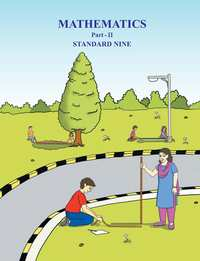Practice Set 5.1Practice Set 5.2Practice Set 5.3Practice Set 5.4Practice Set 5.5Problem Set 5

### Balbharati solutions for Maharashtra state board SSC 9th Standard Maths 2 Geometry Chapter 5 Quadrilaterals Practice Set 5.1 [Page 62]

Practice Set 5.1 | Q 1 | Page 62

Diagonals of a parallelogram WXYZ intersect each other at point O. If ∠XYZ = 135°  then what is the measure of  ∠XWZ and  ∠YZW ?
If l(OY)= 5 cm then l(WY)= ?

Practice Set 5.1 | Q 2 | Page 62

In a parallelogram ABCD, If ∠A =(3x + 2)° , ∠B = (2x - 32)° then find the value of x and then find the measures of  ∠C and  ∠D.

Practice Set 5.1 | Q 3 | Page 62

Perimeter of a parallelogram is 150 cm. One of its sides is greater than the other side by 25 cm. Find the lengths of all sides.

Practice Set 5.1 | Q 4 | Page 62

If the ratio of measures of two adjacent angles of a parallelogram is 1 : 2, find the measures of all angles of the parallelogram.

Practice Set 5.1 | Q 5 | Page 62

Diagonals of a parallelogram intersect each other at point O. If AO = 5, BO = 12 and AB = 13 then show that
squareABCD is a rhombus.

Practice Set 5.1 | Q 6 | Page 62

In the given figure, square PQRS and square ABCR are two parallelograms. If  ∠P =110°  then find the measures of  all angles of square ABCR.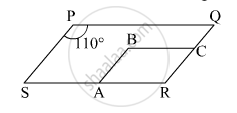Practice Set 5.1 | Q 7 | Page 62

In the given figure, square ABCD is a parallelogram. Point E is on the ray AB such that BE = AB then prove that line ED bisects seg BC at point F.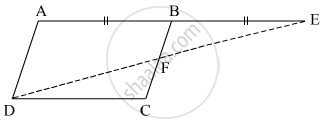### Balbharati solutions for Maharashtra state board SSC 9th Standard Maths 2 Geometry Chapter 5 Quadrilaterals Practice Set 5.2 [Page 67]

Practice Set 5.2 | Q 1 | Page 67

square ABCD is a parallelogram, P and Q are midpoints of side AB and DC respectively, then prove square APCQ is a parallelogram.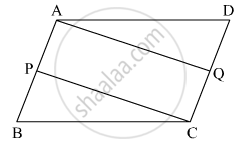Practice Set 5.2 | Q 2 | Page 67

Using opposite angles test for parallelogram, prove that every rectangle is a parallelogram.

Practice Set 5.2 | Q 3 | Page 67

In the given figure, G is the point of concurrence of medians of Δ DEF. Take point H on ray DG such that D-G-H and DG = GH, then prove that square GEHF is a parallelogram.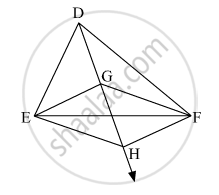Practice Set 5.2 | Q 4 | Page 67

Prove that quadrilateral formed by the intersection of angle bisectors of all angles of a parallelogram is a rectangle.(shown in the given figure)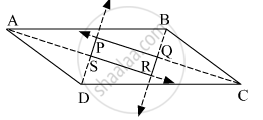Practice Set 5.2 | Q 5 | Page 67

In the given figure, if points P, Q, R, S are on the sides of parallelogram such that AP = BQ = CR = DS then prove that square PQRS is a parallelogram.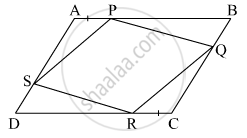### Balbharati solutions for Maharashtra state board SSC 9th Standard Maths 2 Geometry Chapter 5 Quadrilaterals Practice Set 5.3 [Page 69]

Practice Set 5.3 | Q 1 | Page 69

Diagonals of a rectangle ABCD intersect at point O. If AC = 8 cm then find BO and if ∠CAD =35°  then find ∠ACB

Practice Set 5.3 | Q 2 | Page 69

In a rhombus PQRS if PQ = 7.5 then find QR. If ∠QPS = 75° then find the measure of ∠PQR and ∠SRQ.

Practice Set 5.3 | Q 3 | Page 69

Diagonals of a square IJKL intersects at point M, Find the measures of ∠ IMJ, ∠ JIK and ∠ LJK .

Practice Set 5.3 | Q 4 | Page 69

Diagonals of a rhombus are 20 cm and 21 cm respectively, then find the side of rhombus and its perimeter.

Practice Set 5.3 | Q 5.1 | Page 69

State with reason whether the following statement is ‘true’ or ‘false’.

Every parallelogram is a rhombus.

Practice Set 5.3 | Q 5.2 | Page 69

State with reason whether the following statement is  ‘true’ or ‘false’.

Every rhombus is a rectangle.

Practice Set 5.3 | Q 5.3 | Page 69

State with Reason Whether the Following Statement is ‘True’ Or ‘False’.

Every rectangle is a parallelogram.

Practice Set 5.3 | Q 5.4 | Page 69

State with reason whether the following statement is  ‘true’ or ‘false’.

Every squre is a rectangle.

Practice Set 5.3 | Q 5.5 | Page 69

State with reason whether the following statement is  ‘true’ or ‘false’.

Every square is a rhombus.

Practice Set 5.3 | Q 5.6 | Page 69

State with reason whether the following statement is ‘true’ or ‘false’.

Every parallelogram is a rectangle.

### Balbharati solutions for Maharashtra state board SSC 9th Standard Maths 2 Geometry Chapter 5 Quadrilaterals Practice Set 5.4 [Page 71]

Practice Set 5.4 | Q 1 | Page 71

In square IJKL,  side IJ || side KL ∠I =180° ∠K = 53° then find the measure of ∠J and  ∠L.

Practice Set 5.4 | Q 2 | Page 71

In square ABCD , side  BC || side AD, side AB ≅ side DC  If ∠A = 72°  then find the measure of  ∠ B, and  ∠ D.

Practice Set 5.4 | Q 3 | Page 71

In squareABCD , side BC < side AD in following figure .
side BC || side AD and if side BA ≅ side CD then prove that ∠ ABC ≅ ∠ DCB.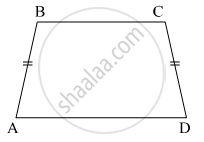### Balbharati solutions for Maharashtra state board SSC 9th Standard Maths 2 Geometry Chapter 5 Quadrilaterals Practice Set 5.5 [Page 73]

Practice Set 5.5 | Q 1 | Page 73

In the given figure, points X, Y, Z are the midpoints of side AB, side BC and side AC of Δ ABC respectively. AB = 5 cm, AC = 9 cm and BC = 11 cm. Find the length of XY, YZ, XZ.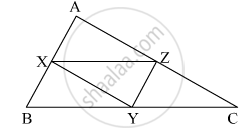Practice Set 5.5 | Q 2 | Page 73

In the given figure, square PQRS and square MNRL are rectangles. If point M is the midpoint of side PR then prove that,

(i) SL = LR,    (ii) LN = 1/2SQ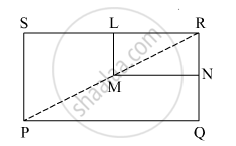Practice Set 5.5 | Q 3 | Page 73

In the given figure, Δ ABC is an equilateral traingle. Points F,D and E are midpoints of side AB, side BC, side AC respectively. Show that Δ FED is an equilateral traingle.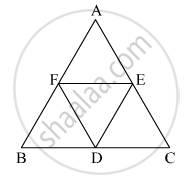Practice Set 5.5 | Q 4 | Page 73

In the given figure, seg PD is a median of Δ PQR. Point T is the mid point of seg PD. Produced QT intersects PR at M. Show that (PM)/(PR) = 1/3
[Hint: DN || QM]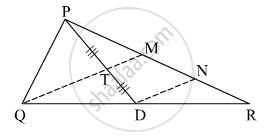### Balbharati solutions for Maharashtra state board SSC 9th Standard Maths 2 Geometry Chapter 5 Quadrilaterals Problem Set 5 [Pages 73 - 74]

Problem Set 5 | Q 1.1 | Page 73

Choose the correct alternative answer and fill in the blank.

If all pairs of adjacent sides of a quadrilateral are congruent then it is called ....

• rectangle

• parallelogram

•  trapezium

• rhombus

Problem Set 5 | Q 1.2 | Page 73

Choose the correct alternative answer and fill in the blank.

If the diagonal of a square is 12 sqrt 2 cm then the perimeter of square is ......

• 24 cm

• 24sqrt2 cm

• 48 cm

• 48 sqrt2 cm

Problem Set 5 | Q 1.3 | Page 73

Choose the correct alternative answer and fill in the blank.

If opposite angles of a rhombus are (2x)° and (3x - 40)° then value of x is ...

• 100°

• 80°

• 160°

• 40°

Problem Set 5 | Q 2 | Page 74

Adjacent sides of a rectangle are 7 cm and 24 cm. Find the length of its diagonal.

Problem Set 5 | Q 3 | Page 74

If diagonal of a square is 13 cm then find its side.

Problem Set 5 | Q 4 | Page 74

Ratio of two adjacent sides of a parallelogram is 3 : 4, and its perimeter is 112 cm. Find the length of its each side.

Problem Set 5 | Q 5 | Page 74

Diagonals PR and QS of a rhombus PQRS are 20 cm and 48 cm respectively. Find the length of side PQ.

Problem Set 5 | Q 6 | Page 74

Diagonals of a rectangle PQRS are intersecting in point M. If  ∠QMR = 50° find the measure of ∠MPS.

Problem Set 5 | Q 7 | Page 74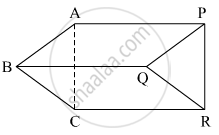if seg AB || seg PQ , seg AB ≅ seg PQ,  seg AC || seg PR, seg AC ≅ seg PR then prove that, seg BC || seg QR and seg BC ≅ seg QR.

Problem Set 5 | Q 8 | Page 74

In the given Figure, square ABCD is a trapezium. AB || DC .Points P and Q are midpoints of seg AD and seg BC respectively.
Then prove that, PQ || AB and PQ = 1/2 (AB + DC).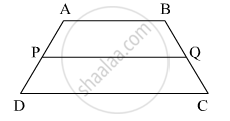Problem Set 5 | Q 9 | Page 74

In the adjacent figure, square ABCD is a trapezium AB || DC . Points M and N are midpoints of diagonal AC and DB respectively then prove that MN || AB .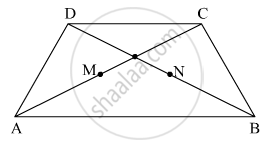Practice Set 5.1Practice Set 5.2Practice Set 5.3Practice Set 5.4Practice Set 5.5Problem Set 5## Balbharati solutions for Maharashtra state board SSC 9th Standard Maths 2 Geometry chapter 5 - Quadrilaterals

Balbharati solutions for Maharashtra state board SSC 9th Standard Maths 2 Geometry chapter 5 (Quadrilaterals) include all questions with solution and detail explanation. This will clear students doubts about any question and improve application skills while preparing for board exams. The detailed, step-by-step solutions will help you understand the concepts better and clear your confusions, if any. Shaalaa.com has the Maharashtra State Board Maharashtra state board SSC 9th Standard Maths 2 Geometry solutions in a manner that help students grasp basic concepts better and faster.

Further, we at Shaalaa.com provide such solutions so that students can prepare for written exams. Balbharati textbook solutions can be a core help for self-study and acts as a perfect self-help guidance for students.

Concepts covered in Maharashtra state board SSC 9th Standard Maths 2 Geometry chapter 5 Quadrilaterals are Concept of Quadrilaterals, Parallelogram, Tests for parallelogram, Properties of Rhombus, Properties of Square, Properties of Rectangle, Concept of Trapezium, The Mid-point Theorem.

Using Balbharati Class 9 solutions Quadrilaterals exercise by students are an easy way to prepare for the exams, as they involve solutions arranged chapter-wise also page wise. The questions involved in Balbharati Solutions are important questions that can be asked in the final exam. Maximum students of Maharashtra State Board Class 9 prefer Balbharati Textbook Solutions to score more in exam.

Get the free view of chapter 5 Quadrilaterals Class 9 extra questions for Maharashtra state board SSC 9th Standard Maths 2 Geometry and can use Shaalaa.com to keep it handy for your exam preparation